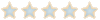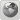NYSMITH MATHCOUNTS COMMUNITY
Would you like to react to this message? Create an account in a few clicks or log in to continue.

# How to easily square numbers ending in 5##How to easily square numbers ending in 5

The following is an easy method to square numbers with a units digit of 5.

1. Begin with the multiplication of 65*65 or something similar
2. Next add one to the tens digit of the number being squared: 6+1=7
3. Multiply the result with the original number: 6*7= 42
4. Place this number before 25 to get 4225
5. This process should work each time

The algebra behind this is as follows:
10X+5 should be represent any number with a units digit of 5.

(10X+5)(10X+5)
100X^2 + 100X + 25
(10X (10X+10)) + 25
((10X)*(10X)(X+1)) +25

((10X)*(10X)(X+1)) shows step , where the original tens digit (10X) is multiplied by the result of step , (10X)(X+1). Step  is represented by the last constant of +25 in the above expression.ArchisPosts : 78
Points : 38
Reputation : 4
Join date : 2008-09-29Permissions in this forum:
You cannot reply to topics in this forum